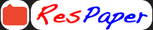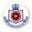Trending ▼   ResFinder# ISC Class XII Prelims 2019 : Mathematics (Alexandra School, Amritsar)

4 pages, 54 questions, 37 questions with responses, 39 total responses,20Sulabh Mehta Alexandra School, Amritsar
+Fave Message
 Home > sulabh56 >   F Also featured on: School Page iscFormatting page ...

L Pre Co~ncil ExaDJinatton - 2019 ClassXII . Time :-3hrs M.l\1. 100 Mathematics AL-50 Candidate are al/owed additional 15 n,i,mtesfor 011/y reading the paper They must 11ot start writing during the time. SECTION A (80 MARKS) 1. (i) Find the identity element in set of all ralional numbers except - 1 with respect to * defined by a b = a + b + nb. 1 a b+c (iQ \$how that : 1 b c + a = 0. 1 c a+b (iii) If sin ( cos- 1 ~ + sin- 1 1 x) = 1, find the value of x. (iv) Find X and Y, if X+ Y= G~] and X - Y = [~ 11 ~ Evaluate: f 1 + ~cotx . 0 (vi) If y = 2 esin x , find ~~ . (viz) Salve the differential equation : dy - e-' Y = e-v - Y. . dx (viii)Prove that the function j (x) = x3 - 6x2 + l2x - 18 is increasing on R. (ix) The probability that a boy will not pass M .B.A examination is ~ and that a girl . 5 will not pass is i . Calculate the probability that atleast one of them passes the examination. 5 (x) If A and B are two events such that P(A) = 1 , P(B) = 4 1 2 and P(A P(not A and not B). 2 2. If f(x) = 3 x- , prove that / /j(x)l 2x-3 = x for all (!: sing properties of determinants, prove that n - . B) = ]_, find 8 [lQ X 2) (4) x e R - {~} . 2 b2 + c2 a2 a2 b2 c2+a2 b2 c2 c2 a2 +b2- = 4a2 b2c2 [4)Formatting page ...

Top Contributors
to this ResPaperPrincess Dragun...(31)Indrani Adhikari(5)Anik Das(4)Anurag Munshi(2)Formatting page ...Formatting page ...